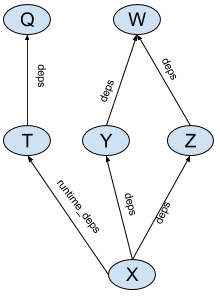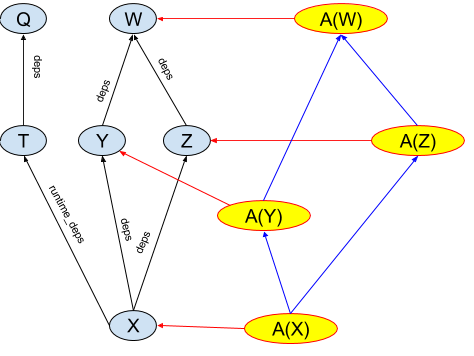# Aspects

Aspects allow augmenting build dependency graphs with additional information and actions. Some typical scenarios when aspects can be useful:

• IDEs that integrate Bazel can use aspects to collect information about the project.
• Code generation tools can leverage aspects to execute on their inputs in “target-agnostic” manner. As an example, BUILD files can specify a hierarchy of protobuf library definitions, and language-specific rules can use aspects to attach actions generating protobuf support code for a particular language.

## Aspect basics

Bazel BUILD files provide a description of a project’s source code: what source files are part of the project, what artifacts (targets) should be built from those files, what the dependencies between those files are, etc. Bazel uses this information to perform a build, that is, it figures out the set of actions needed to produce the artifacts (such as running compiler or linker) and executes those actions. Bazel accomplishes this by constructing a dependency graph between targets and visiting this graph to collect those actions.

Consider the following BUILD file:

``````java_library(name = 'W', ...)
java_library(name = 'Y', deps = [':W'], ...)
java_library(name = 'Z', deps = [':W'], ...)
java_library(name = 'Q', ...)
java_library(name = 'T', deps = [':Q'], ...)
java_library(name = 'X', deps = [':Y',':Z'], runtime_deps = [':T'], ...)
``````

This BUILD file defines a dependency graph shown in the following figure:Bazel analyzes this dependency graph by calling an implementation function of the corresponding rule (in this case “java_library”) for every target in the above example. Rule implementation functions generate actions that build artifacts, such as `.jar` files, and pass information, such as locations and names of those artifacts, to the dependencies of those targets in providers.

Aspects are similar to rules in that they have an implementation function that generates actions and returns providers. However, their power comes from the way the dependency graph is built for them. An aspect has an implementation and a list of all attributes it propagates along. Consider an aspect A that propagates along attributes named “deps”. This aspect can be applied to a target X, yielding an aspect application node A(X). During its application, aspect A is applied recursively to all targets that X refers to in its “deps” attribute (all attributes in A’s propagation list). Thus a single act of applying aspect A to a target X yields a “shadow graph” of the original dependency graph of targets (see Fig.2).The only edges that are shadowed are the edges along the attributes in the propagation set, thus the `runtime_deps` edge is not shadowed in this example. An aspect implementation function is then invoked on all nodes in the shadow graph similar to how rule implementations are invoked on the nodes of the original graph.

## Simple example

This example demonstrates how to recursively print the source files for a rule and all of its dependencies that have a `deps` attribute. It shows an aspect implementation, an aspect definition, and how to invoke the aspect from the Bazel command line.

``````def _print_aspect_impl(target, ctx):
# Make sure the rule has a srcs attribute.
if hasattr(ctx.rule.attr, 'srcs'):
# Iterate through the files that make up the sources and
# print their paths.
for src in ctx.rule.attr.srcs:
for f in src.files.to_list():
print(f.path)
return []

print_aspect = aspect(
implementation = _print_aspect_impl,
attr_aspects = ['deps'],
)
``````

Let’s break the example up into its parts and examine each one individually.

### Aspect definition

``````print_aspect = aspect(
implementation = _print_aspect_impl,
attr_aspects = ['deps'],
)
``````

Aspect definitions are similar to rule definitions, and defined using the `aspect` function.

Just like a rule, an aspect has an implementation function which in this case is `_print_aspect_impl`.

`attr_aspects` is a list of rule attributes along which the aspect propagates. In this case, the aspect will propagate along the `deps` attribute of the rules that it is applied to.

Another common argument for `attr_aspects` is `['*']` which would propagate the aspect to all attributes of a rule.

### Aspect implementation

``````def _print_aspect_impl(target, ctx):
# Make sure the rule has a srcs attribute.
if hasattr(ctx.rule.attr, 'srcs'):
# Iterate through the files that make up the sources and
# print their paths.
for src in ctx.rule.attr.srcs:
for f in src.files.to_list():
print(f.path)
return []
``````

Aspect implementation functions are similar to the rule implementation functions. They return providers, can generate actions, and take two arguments:

• `target`: the target the aspect is being applied to.
• `ctx`: `ctx` object that can be used to access attributes and generate outputs and actions.

The implementation function can access the attributes of the target rule via `ctx.rule.attr`. It can examine providers that are provided by the target to which it is applied (via the `target` argument).

Aspects are required to return a list of providers. In this example, the aspect does not provide anything, so it returns an empty list.

### Invoking the aspect using the command line

The simplest way to apply an aspect is from the command line using the `--aspects` argument. Assuming the rule above were defined in a file named `print.bzl` this:

``````bazel build //MyExample:example --aspects print.bzl%print_aspect
``````

would apply the `print_aspect` to the target `example` and all of the target rules that are accessible recursively via the `deps` attribute.

The `--aspects` flag takes one argument, which is a specification of the aspect in the format `<extension file label>%<aspect top-level name>`.

The following example demonstrates using an aspect from a target rule that counts files in targets, potentially filtering them by extension. It shows how to use a provider to return values, how to use parameters to pass an argument into an aspect implementation, and how to invoke an aspect from a rule.

FileCount.bzl file:

``````FileCountInfo = provider(
fields = {
'count' : 'number of files'
}
)

def _file_count_aspect_impl(target, ctx):
count = 0
# Make sure the rule has a srcs attribute.
if hasattr(ctx.rule.attr, 'srcs'):
# Iterate through the sources counting files
for src in ctx.rule.attr.srcs:
for f in src.files.to_list():
if ctx.attr.extension == '*' or ctx.attr.extension == f.extension:
count = count + 1
# Get the counts from our dependencies.
for dep in ctx.rule.attr.deps:
count = count + dep[FileCountInfo].count
return [FileCountInfo(count = count)]

file_count_aspect = aspect(implementation = _file_count_aspect_impl,
attr_aspects = ['deps'],
attrs = {
'extension' : attr.string(values = ['*', 'h', 'cc']),
}
)

def _file_count_rule_impl(ctx):
for dep in ctx.attr.deps:
print(dep[FileCountInfo].count)

file_count_rule = rule(
implementation = _file_count_rule_impl,
attrs = {
'deps' : attr.label_list(aspects = [file_count_aspect]),
'extension' : attr.string(default = '*'),
},
)
``````

BUILD.bazel file:

``````load('//file_count.bzl', 'file_count_rule')

cc_library(
name = 'lib',
srcs = [
'lib.h',
'lib.cc',
],
)

cc_binary(
name = 'app',
srcs = [
'app.h',
'app.cc',
'main.cc',
],
deps = ['lib'],
)

file_count_rule(
name = 'file_count',
deps = ['app'],
extension = 'h',
)
``````

### Aspect definition

``````file_count_aspect = aspect(implementation = _file_count_aspect_impl,
attr_aspects = ['deps'],
attrs = {
'extension' : attr.string(values = ['*', 'h', 'cc']),
}
)
``````

In this example, we are again propagating the aspect via the `deps` attribute.

`attrs` defines a set of attributes for an aspect. Public aspect attributes are of type `string` and are called parameters. Parameters must have a`values` attribute specified on them. In this case we have a parameter called `extension` that is allowed to have ‘`*`’, ‘`h`’, or ‘`cc`’ as a value.

Parameter values for the aspect are taken from the string attribute with the same name of the rule requesting the aspect (see the definition of `file_count_rule`). Aspects with parameters cannot be used via the command line because there is no syntax to define the parameters.

Aspects are also allowed to have private attributes of types `label` or `label_list`. Private label attributes can be used to specify dependencies on tools or libraries that are needed for actions generated by aspects. There is not a private attribute defined in this example, but the following code snippet demonstrates how you could pass in a tool to a aspect:

``````...
attrs = {
'_protoc' : attr.label(
default = Label('//tools:protoc'),
executable = True,
cfg = "host"
)
}
...
``````

### Aspect implementation

``````FileCountInfo = provider(
fields = {
'count' : 'number of files'
}
)

def _file_count_aspect_impl(target, ctx):
count = 0
# Make sure the rule has a srcs attribute.
if hasattr(ctx.rule.attr, 'srcs'):
# Iterate through the sources counting files
for src in ctx.rule.attr.srcs:
for f in src.files.to_list():
if ctx.attr.extension == '*' or ctx.attr.extension == f.extension:
count = count + 1
# Get the counts from our dependencies.
for dep in ctx.rule.attr.deps:
count = count + dep[FileCountInfo].count
return [FileCountInfo(count = count)]
``````

Just like a rule implementation function, an aspect implementation function returns a struct of providers that are accessible to its dependencies.

In this example, the `FileCountInfo` is defined as a provider that has one field `count`. It is best practice to explicitly define the fields of a provider using the `fields` attribute.

The set of providers for an aspect application A(X) is the union of providers that come from the implementation of a rule for target X and from the implementation of aspect A. It is an error if a target and an aspect that is applied to it each provide a provider with the same name. The providers that a rule implementation propagates are created and frozen before aspects are applied and cannot be modified from an aspect.

The parameters and private attributes are passed in the attributes of the `ctx`. In this example, we reference the `extension` parameter to decide what files to count.

For returning providers, the values of attributes along which the aspect is propagated (from the `attr_aspect` list) are replaced with the results of an application of the aspect to them. For example, if target X has Y and Z in its deps, `ctx.rule.attr.deps` for A(X) will be [A(Y), A(Z)]. In this example, `ctx.rule.attr.deps` are Target objects that are the results of applying the aspect to the ‘deps’ of the original target to which the aspect has been applied.

In the example, the aspect accesses the `FileCountInfo` provider from the target’s dependencies to accumulate the total transitive number of files.

### Invoking the aspect from a rule

``````def _file_count_rule_impl(ctx):
for dep in ctx.attr.deps:
print(dep[FileCountInfo].count)

file_count_rule = rule(
implementation = _file_count_rule_impl,
attrs = {
'deps' : attr.label_list(aspects = [file_count_aspect]),
'extension' : attr.string(default = '*'),
},
)
``````

The rule implementation demonstrates how to access the `FileCountInfo` via the `ctx.attr.deps`.

The rule definition demonstrates how to define a parameter (`extension`) and give it a default value (`*`). Note that having a default value that was not one of ‘`cc`’, ‘`h`’, or ‘`*`’ would be an error due to the restrictions placed on the parameter in the aspect definition.

### Invoking an aspect through a target rule

``````load('//file_count.bzl', 'file_count_rule')

cc_binary(
name = 'app',
...
)

file_count_rule(
name = 'file_count',
deps = ['app'],
extension = 'h',
)
``````

This demonstrates how to pass the `extension` parameter into the aspect via the rule. Since the `extension` parameter has a default value in the rule implementation, `extension` would be considered an optional parameter.

When the `file_count` target is built, our aspect will be evaluated for itself, and all of the targets accessible recursively via `deps`.